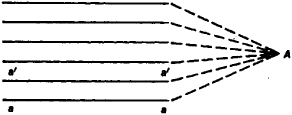# Elements at Infinity

## Elements at Infinity

in mathematics, those elements—called points, lines, planes—which are added to the Euclidean plane or Euclidean space to make possible the interpretation of certain branches of mathematics—projective geometry, the theory of functions of a complex variable, and other branches.

The origin of the term “element at infinity” is most easily deduced from the following example. Let us consider the parallel lines a and a' in the Euclidean plane a (Figure 1) and the straight line b, which intersects them at points M and M', respectively. We will rotate line b around point M' in the direction indicated by the arrow in the figure until it coincides with line a'. It is evident that as line b approaches line a', point M of intersection of lines a and b will move away to infinity. This process explains clearly enough the often used expression “parallel straight lines intersect at a point at infinity.”

The above visual presentations form the basis of the interpretation of two-dimensional projective geometry on the Euclidean plane ɑ. For this purpose, points at infinity andFigure 1

one line at infinity are added in the following manner to the plane α It is agreed to consider parallel lines as intersecting at a point at infinity. Then line a', which is parallel to line a (Figure 2), intersects it at some point; however, this point is not an ordinary point but a new concept, a point at infinity of line a. It is agreed that all lines parallel to line a have one common point at infinity A, and the points at infinity of non-parallel lines are considered distinct. Thus, the Euclidean plane is enlarged by an infinite number of points at infinity. The set of all these points at infinity of the plane α is called a line at infinity.Figure 2

The plane α, enlarged in this manner by points at infinity and a line at infinity, is called a projective plane. Its properties differ from those of the Euclidean plane—for example, in a projective plane any two lines intersect.

The Euclidean plane can be enlarged by elements at infinity and by other methods. Thus, in the representation of complex numbers on the Euclidean plane, the latter is enlarged by one point at infinity which corresponds to one infinitely large complex number.

### REFERENCE

Efimov, N. V. Vysshaia geometriia, 4th ed. Moscow, 1961.

E. G. POZNIAK

Site: Follow: Share:
Open / Close